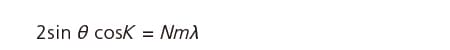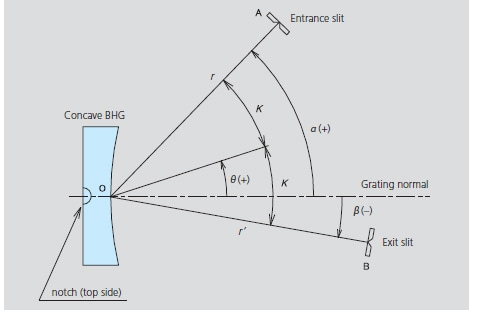# Diffraction GratingsConcave Blazed Holographic Gratings

Constant-deviation monochromators are compact spectrometers that allow wavelength scanning to be performed with fixed entrance and exit slits simply by rotating the grating. In general, the angle formed by the incident light and the diffracted light is called the "deviation angle." As shown in Fig. 32, if O, A, and B represent the center of the grating, the entrance slit, and the exit slit respectively, then ∠AOB is a fixed angle, which is expressed as "2K." If θ is the rotation angle of the grating, then the incident angle α, the diffraction angle β, and 2K satisfy the following: 2K = α - β = constant, α = K + θ , and β = θ - K. Here, α, β, and θ are the angles made with normal to the grating and the counterclockwise direction is taken as positive. Grating equation (8) gives the following:

#### (25)It can be seen from this that wavelength scanning can be performed just by changing rotation angle θ.Fig.4 Concave BHG Mounting for Constant-deviation Monochromator

### The various parameters are explained below.

#### Groove density N :

The groove density at the center of the concave grating.

#### Wavelength range λ 1 to λ 2 :

The optimum wavelength range for the Shimadzu design. Outside this range, aberrations become larger and imaging performance deteriorates. λ 1 and λ 2 respectively represent the minimum and maximum wavelengths in this range.

#### Blaze wavelength λ B :

The blaze wavelength λ B for the mounting parameters and not the blaze wavelength for the Littrow mounting λ B(Litt). Refer to Blaze Wavelength under Overview of Gratings for details.

#### Dimensions W, H, and T :

"W" is the width perpendicular to the grooves. "H" is the height parallel to the grooves.
"T" is the thickness at the center. (Refer to Fig.3)

#### Code number :

Specify a code number when ordering.

### Table 4 Standard Aberration-corrected Concave Gratings for Constant-deviation Monochromators

• *1 : Nominal blaze wavelength is the wavelength of the maximum diffraction efficiency (target) with the design mounting.
No one to guarantee the wavelength of the maximum diffraction efficiency.
Blazing is performed with an ion-beam etching technology.
• *2 : Relative efficiency minimum is at the nominal blaze wavelength.
In the interests of product improvement, the external appearance and specifications are subject to change without notice.